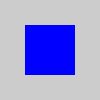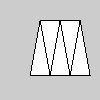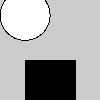Name

### createShape()

Examples```size(100, 100, P2D)
# Creating the PShape as a square. The
# numeric arguments are similar to rect().
square = createShape(RECT, 0, 0, 50, 50)
square.setFill(color(0, 0, 255))
square.setStroke(False)
shape(square, 25, 25)
``````size(100, 100, P2D)
# Creating a custom PShape as a square, by
# specifying a series of vertices.
s = createShape()
s.beginShape()
s.fill(0, 0, 255)
s.noStroke()
s.vertex(0, 0)
s.vertex(0, 50)
s.vertex(50, 50)
s.vertex(50, 0)
s.endShape(CLOSE)
shape(s, 25, 25)
``````size(100, 100, P2D)
s = createShape()
s.beginShape(TRIANGLE_STRIP)
s.vertex(30, 75)
s.vertex(40, 20)
s.vertex(50, 75)
s.vertex(60, 20)
s.vertex(70, 75)
s.vertex(80, 20)
s.vertex(90, 75)
s.endShape()
shape(s, 0, 0)
``````size(100, 100, P2D)
# Create the shape group
alien = createShape(GROUP)
# Make two shapes
head = createShape(ELLIPSE, -25, 0, 50, 50)
body = createShape(RECT, -25, 45, 50, 40)
body.setFill(color(0))
# Add the two "child" shapes to the parent group

background(204)
translate(50, 15)
shape(alien)  # Draw the group
```
Description The createShape() function is used to define a new shape. Once created, this shape can be drawn with the shape() function. The basic way to use the function defines new primitive shapes. One of the following parameters are used as the first parameter: ELLIPSE, RECT, ARC, TRIANGLE, SPHERE, BOX, QUAD, LINE. The parameters for each of these different shapes are the same as their corrsponding functions: ellipse(), rect(), arc(), triangle(), sphere(), box(), and line(). The first example above clarifies how this works.

Custom, unique shapes can be made by using createShape() without a parameter. After the shape is started, the drawing attributes and geometry can be set directly to the shape within the beginShape() and endShape() methods. See the second example above for specifics.

Geometry that groups vertices to build larger forms, such as group of triangles, can be created with parameters to beginShape(). These options are POINTS, LINES, TRIANGLES, TRIANGLE_FAN, TRIANGLE_STRIP, QUADS, and QUAD_STRIP. See the third example above.

The createShape() function can also be used to make a complex shape made of other shapes. This is called a "group" and it's created by using the parameter GROUP as the first parameter. See the fourth example above to see how it works.

When a shape is first created inside the beingShape() and endShape() methods, the normal Processing style functions like fill() and stroke() are used to define the drawing attributes. However, after a shape is created, a different set of functions needs to be used. These include the setFill() and setStroke() functions shown in the examples above. The complete list of methods and fields for the PShape class are in the Processing Javadoc.
Related PShape
endShape()